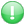Get premium membership and access revision papers, questions with answers as well as video lessons.

# Econ 100:Introduction To Economics Question Paper

Econ 100:Introduction To Economics

Course:Bachelor Of Science

Institution: Laikipia University question papers

Exam Year:2016

INSTRUCTIONS: Answer question one and any other two questions
QUESTION ONE
a)Explain the following economic concepts:
1. Microeconomics and macroeconomics (2 marks)
2. Gross Domestic Product (G.D.P) and Gross National Product (G.N.P) (2 marks)
3. Consumer surplus (2 marks)
4. Economics of scale and diseconomies of scale (2 marks)
5. Indifference curve and an isoquant (2 marks)
b) Economics is the study of scarcity and choice. Examine why the science of economics suites this definition.(10 marks)
c) Explain what is meant by consumer sovereignity. What are the major limitations on the sovereignty of the consumer? (10marks)
QUESTION TWO
a) What do you understand by production function? Explain factors that affect it in an economy (10 marks)
(b) Explain the term National Income and National Income accounting of a country.(2 marks)
c) Explain the three methods used in its measurement.(8 marks)
QUESTION THREE
a) Write explanatory notes on the following concepts.
1.Utility and satisfaction (2 marks)
2.Inflations and deflations (2 marks)
3. The circular flow of National Income (2 marks)
4. Economic growth and Economic Development (2 marks)
5.The law of Demand and the law of supply (2 marks)
QUESTION FOUR
a) Explain why inflation and unemployment are o economic concern (10marks)
b) Why is resources Devolution essential in a country like Kenya? (10 marks)